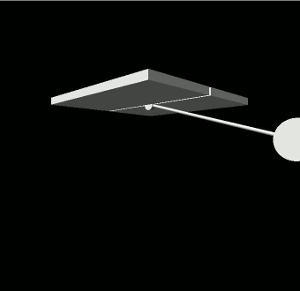# Fancy Simple Pendulum in Visual Python

Here is the visual python code for a “fancy” pendulum exhibiting simple harmonic motion. Clicking anywhere in the window will reset the pendulum to that angle.```
from __future__ import division, print_function
from visual import *
import numpy as np

# Set the constants
g = 9.80            # (m/s^2)
L = 10              # Length of the pendulum
initialAngle = 1.3  # In radians

# Make the pendulum bob, a small ceiling, a hinge, and the rod.
position = [L*np.sin(initialAngle), -L*np.cos(initialAngle), -0.15]
pend = cylinder(pos=position, axis=(0, 0, 0.3), radius=1)
box(pos=(0, 0.25, -2.6), length=10, height=0.5, width=5)
box(pos=(0, 0.25, 2.6), length=10, height=0.5, width=5)
cylinder(pos=(0, 0, -0.15), axis=(0, 0, 0.3), radius=0.4)
rod = cylinder(pos=(0, 0, 0), axis=(pend.pos, pend.pos, 0), radius=0.1)

def position(time):
"""
Given time, t, this function moves the pendulum to its new position. We
use the equation:
theta(t) = theta_0*cos(sqrt(g/L)*t)
"""
theta = initialAngle*np.cos((g/L)**(1/2)*time)          # Angle of the pendulum
pend.pos = [L*np.sin(theta), -L * np.cos(theta), -0.15] # Update position of bob
rod.axis = [pend.pos, pend.pos, 0]                # Update rod's position

i = 0
while True:
rate(100)
if scene.mouse.clicked:
m = scene.mouse.getclick()
angle = np.arctan2(m.pos, -m.pos)
pend.pos = [L * np.sin(angle), -L * np.cos(angle), -0.15]
rod.axis = [pend.pos, pend.pos, 0]
initialAngle = angle
else:
position(i)
i += 0.01

```

## 2 thoughts on “Fancy Simple Pendulum in Visual Python”

1.Anand says:

This script does not work in my anaconda Ver3.7 install under Windows 10.
Does it in anaconda Ver2.7 ? How can we make this work?
But I also have PythonXY() 2.7 and only in that it works

1.leonh says:

I just tested the version at https://github.com/leonhostetler/undergrad-projects/blob/master/computational-physics/04_visual_python/fancy_simple_pendulum.py (which I believe is the same as the above) in my Python 2.7 on Linux, and it works. When I first wrote this, I wrote it on Windows in some version of Python 3, I believe. So it should work provided that you have VPython installed and working.

This site uses Akismet to reduce spam. Learn how your comment data is processed.